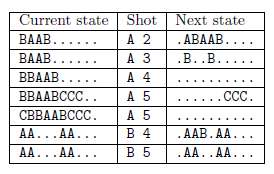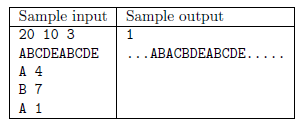Mathematical and Physical Journal
for High Schools
Issued by the MATFUND Foundation
 Already signed up? New to KöMaL?

#Problem I. 382. (October 2015)

I. 382. ZUMA is a shooting game played on various boards. During the game the player should eliminate balls$\displaystyle -$having different colors and rolling along a line initially in one block$\displaystyle -$before any of the balls reach the edge of the board by shooting other balls at them. Create your program to simulate the game: the balls should roll along a straight line from left to right, and a shot is fired in each time unit. The detailed rules are as follows:

$\displaystyle \bullet$ initially all balls are located on the left of the board with no gaps between them;

$\displaystyle \bullet$ the first ball from the left is shifted to the right by one unit in each time unit;

$\displaystyle \bullet$ each ball is shifted whose neighbor has been shifted;

$\displaystyle \bullet$ a missile ball shot at the other balls reaches its destination after the shift described above took place, but still in the same time unit

$\displaystyle \circ$ if there is a ball at the impact position, then

$\displaystyle *$ if there is a block of balls adjacent to the impact position and having the same color as the missile color, then

- they disappear, that is, their positions become empty;

- if there are altogether at least 3 balls of the same color on the two sides of this newly created gap, then they also disappear, and this is repeated recursively;

$\displaystyle *$ otherwise the missile ball stays at the impact position, and the ball that was there is shifted to the right, and all balls to the right are shifted whose neighbor has been shifted;

$\displaystyle \circ$ if there is no ball at the impact position, then

$\displaystyle *$ if there is a ball in a neighboring position, then the missile ball stays at the impact position;

$\displaystyle *$ otherwise the missile ball disappears from the impact position.

The first line of the input file contains the length of the board $\displaystyle h$, the number of balls $\displaystyle p$ rolling on the board, and the number of balls $\displaystyle k$ shot during the game. The second line of the input contains $\displaystyle p$ characters (from the set A, ..., F) denoting the ball colors on the board and starting from the left. Then each of the next $\displaystyle k$ lines describes a ball--position pair: the first character denotes the missile ball color, and the second number is the impact position of this ball when it hits the board. The output shows the final state of the system after the last missile ball has been shot.

$\displaystyle \bullet$ If all balls were eliminated by the shots, then the first line of the output should be 0, and the second output line should be the number of the shot which first achieved this.

$\displaystyle \bullet$ If any of the balls reached the edge of the board, then the first output line should be -1, and the second output line should be the number of the shot after which this happened.

$\displaystyle \bullet$ If there is at least one ball on the board but none of them reached the edge of the board, then the first output line should be 1, and the second output line should contain $\displaystyle h$ characters denoting the ball colors still on the board from left to right, and with . denoting an empty position.

The following example shows 7 different and independent board positions, a shot, and the state of the board after this shot.The first and second command-line arguments to your program are the names of the input and output files, respectively.

The source code of your program$\displaystyle -$with a short documentation also describing your solution and specifying the name of the developer environment to use for compiling the source$\displaystyle -$should be submitted in a compressed file i382.zip.

(10 pont)

Deadline expired on November 10, 2015.

### Statistics:

 10 students sent a solution. 8 points: 2 students. 7 points: 2 students. 6 points: 1 student. 5 points: 3 students. 1 point: 2 students.

Problems in Information Technology of KöMaL, October 2015